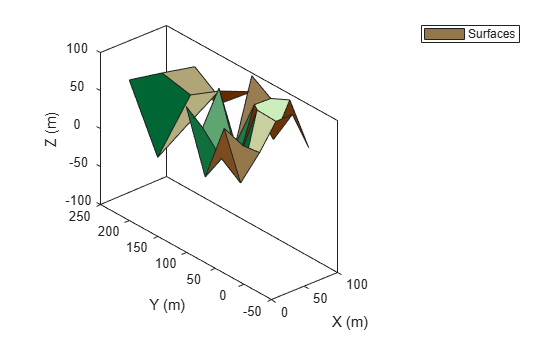# surfacePlotterData

Data for surface plotter

Since R2022b

## Syntax

``plotterData = surfacePlotterData(manager)``
``plotData = surfacePlotterData(___,colorMap)``

## Description

example

````plotterData = surfacePlotterData(manager)` returns a data structure `plotData` that you can use as input to the `plotSurface` function for plotting surfaces managed by the `SurfaceManager` object `manager`.```
````plotData = surfacePlotterData(___,colorMap)` specifies the color map for the plot data.```

## Examples

collapse all

`scenario = radarScenario;`

Define the terrain and boundaries of two surfaces and add the two surfaces to the radar scenario.

```terrain1 = randi(100,4,5); terrain2 = randi(100,3,3); boundary1 = [0 100; 0 100-eps]; boundary2 = [0 100; 100 200]; s1 = landSurface(scenario,Terrain=terrain1,Boundary=boundary1); s2 = landSurface(scenario,Terrain=terrain2,Boundary=boundary2);```

Obtain the plotter data by using the `surfacePlotterData` function.

`plotterData = surfacePlotterData(scenario.SurfaceManager)`
```plotterData=1×2 struct array with fields: X Y Z C ```

Create a `theaterPlot` object and specify the axis limits of the plot.

`theaterpplot = theaterPlot(ZLimits=[-50 150],YLimits=[-50 250],ZLimits=[-100 100]);`

Create a surface plotter.

`plotter = surfacePlotter(theaterpplot,DisplayName="Surfaces");`

Plot surfaces in the theater plot. Change view angles for better visualization.

```plotSurface(plotter,plotterData) view(-41,29)```## Input Arguments

collapse all

Surface manager, specified as a surface manager object.

Color map used for plotting surfaces, specified as a three-column matrix of RGB triplets. An RGB triplet is a three-element row vector whose elements specify the intensities of the red, green, and blue components of the color. The intensities can be double or single values in the range [0, 1], or they can be `uint8` values in the range [0, 255]. For example, this matrix defines a colormap containing five colors:

```map = [0.2 0.1 0.5 0.1 0.5 0.8 0.2 0.7 0.6 0.8 0.7 0.3 0.9 1 0];```

Color`double` or `single` RGB Triplet`uint8` RGB Triplet
yellow`[1 1 0]``[255 255 0]`
magenta`[1 0 1]``[255 0 255]`
cyan`[0 1 1]``[0 255 255]`
red`[1 0 0]``[255 0 0]`
green`[0 1 0]``[0 255 0]`
blue`[0 0 1]``[0 0 255]`
white`[1 1 1]``[255 255 255]`
black`[0 0 0]``[0 0 0]`

## Output Arguments

collapse all

Plotter data, returned as a P-element array of structures, where P is the number of surfaces saved in the `Surfaces` property of the `SurfaceManager` object `manager`. Each structure has these fields:

Field NameDescription
`X`Domain of the surface in the x-direction, returned as an M-element real-valued vector. M is the number of x-coordinates for defining the terrain of the surface.
`Y`Domain of the surface in the y-direction, returned as an N-element real-valued vector. N is the number of y-coordinates for defining the terrain of the surface.
`Z`Height values of the surface, returned as an N-by-M real-valued matrix. N is the number of elements in the `Y` field, and M is the number of elements in the X field.
`C`Color for vertices in the terrain of the surface, returned as an N-by-M-by-3 matrix of RGB triplets. N is the number of elements in the `Y` field, and M is the number of elements in the X field. The `plotSurface` function determines the color of a surface patch based on the color of its first vertex.

## Version History

Introduced in R2022b